Latest Banking jobs   »

# Quantitative Aptitude Quiz For IBPS Clerk Prelims 2022- 7th August

Directions (1-5):- Given line graph shows the sum invested, rate of interest and time period of investment by 4 people. Study the data carefully and answer the questions.
(NOTE: all invested their sum at simple interest)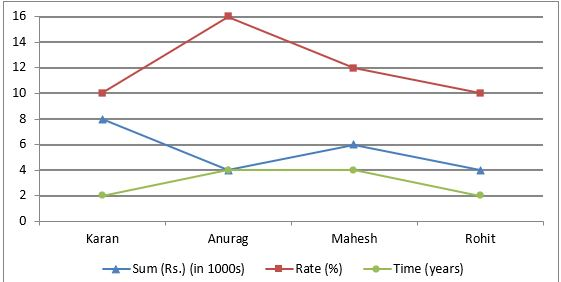Q1. How much will Rohit receive after completion of his investment period? (in Rs.)
(a) 5200
(b) 6800
(c) 4800
(d) 4400
(e) 4600

Q2. Interest amount received by Mahesh is what percent more than interest amount received by Karan?
(a) 85%
(b) 60%
(c) 75%
(d) 70%
(e)80%

Q3. What is total amount received as interest by Anurag & Rohit together? (in Rs.)
(a)None of these
(b) 3150
(c) 3200
(d) 3360
(e) 3420

Q4. If Karan had invested same sum at compound interest at same rate of interest for same period. How much more would he earn?
(a)Rs 80
(b)Rs 90
(c) Rs 70
(d) Rs 60
(e) None of these

Q5. Who among the four had received the highest amount as interest?
(a) Karan
(b) Anurag
(c) Both Anurag & Mahesh
(d) Rohit
(e) Mahesh

Directions (6–10): What will come in the place of question (?) mark in following the question: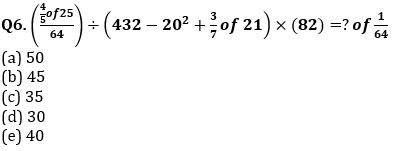Q7. 55% of 900 + 70% of 1050 = ?% of 3000
(a) 41
(b) 42
(c) 43
(d) 44
(e) 45

Q8. 73823-34156+4756+6758-9849=41499-160-?
(a) 5
(b) 7
(c) 4
(d) 8
(e) 6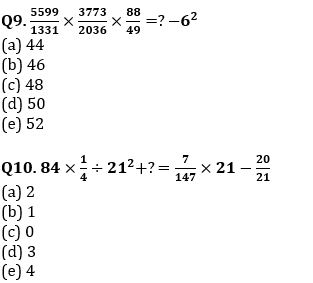Directions (11-15):- In each of the following questions, two equations (I) and (II) are given. Solve the equations and mark the correct option:
(a) if x>y
(b) if x≥y
(c) if x<y
(d) if x ≤y
(e) if x = y or no relation can be established between x and y.

Q11. I. x²+23x+132=0
II. y²+21y+110=0

Q12. I. 3x²+20x+32=0
II. 5y²+23y+24=0

Q13. I. x²-29x+208=0
II. y²-21y+108=0

Q14. I. x²+30x+224=0
II.y²+35y+306=0

Q15. I. x= ∛4096
II. y² =256

Solutions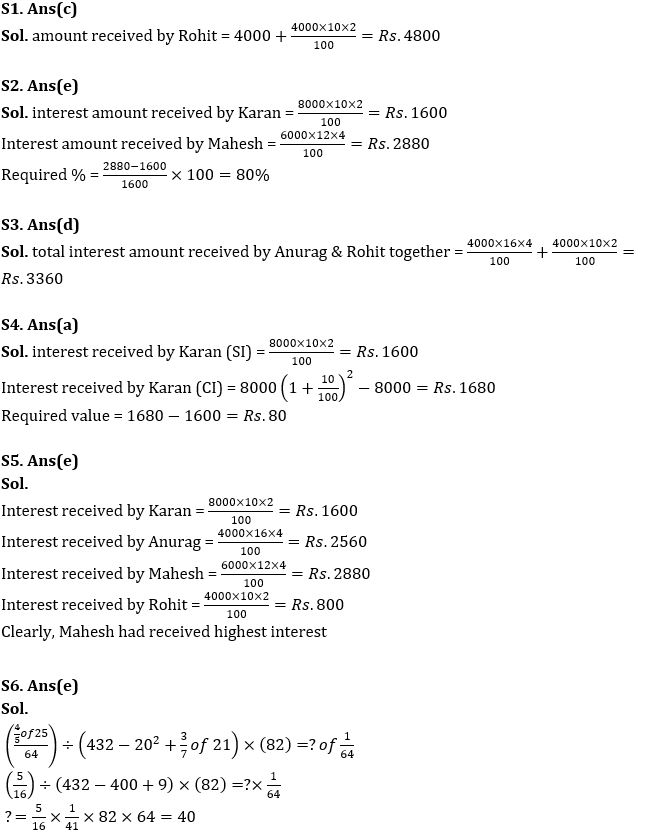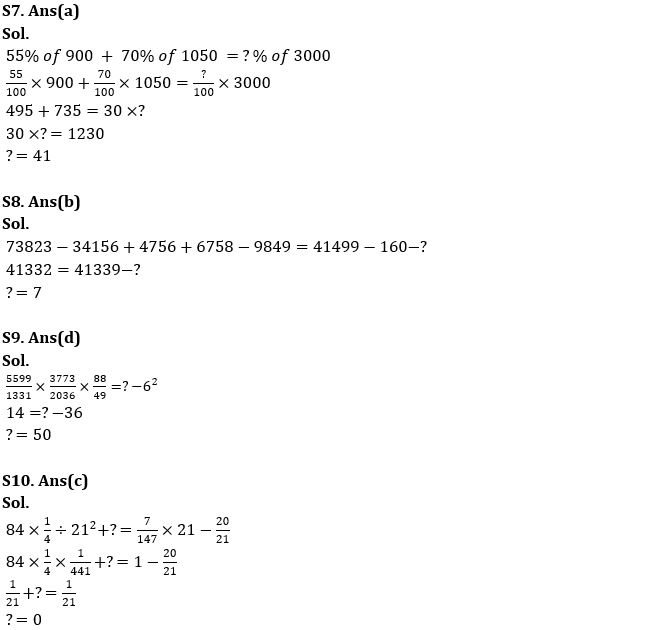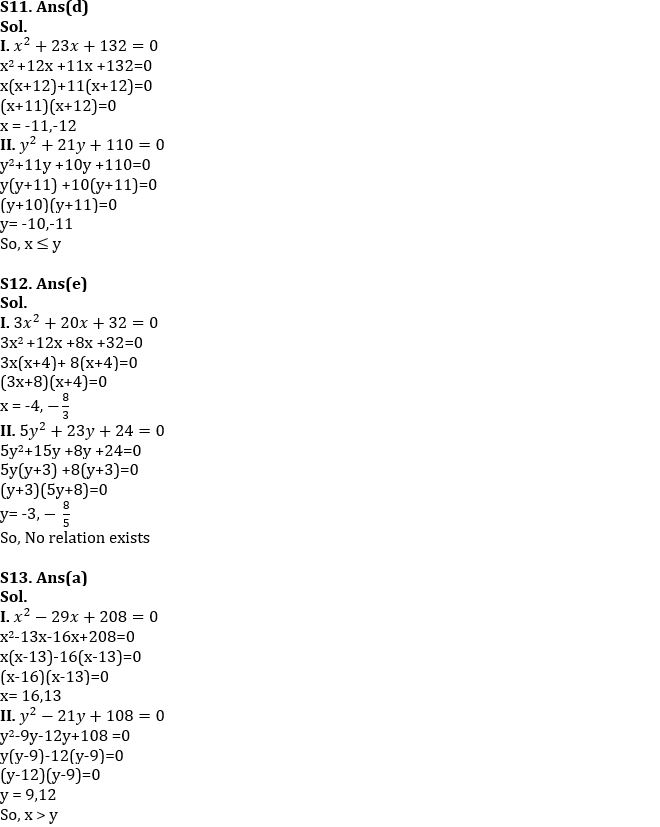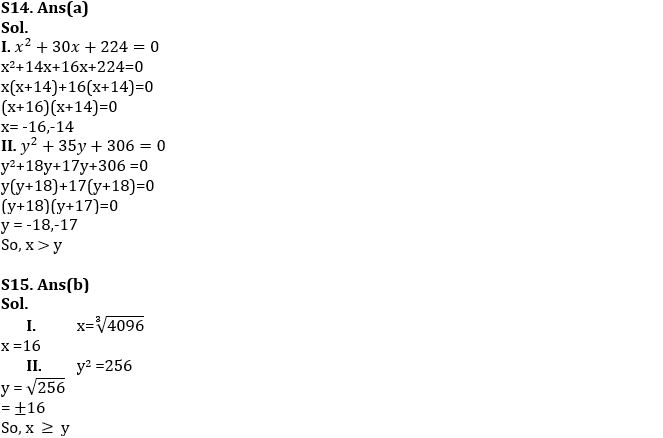#### Congratulations!Download Hindu Review of October 2021: Free PDF